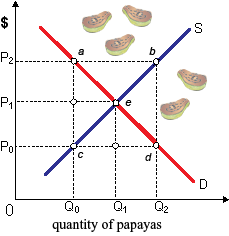Paying bond by given interest rate

When you buy a bond when the interest rate is 10 percent and sell it while the interest rate is 15%, you will obtain: (w) less than you paid for the bond. (x) more than you paid for the bond. (y) identical amount that you paid for the bond. (z) income taxable at higher rates than wages.

Can anybody suggest me the proper explanation for given problem regarding Economics generally?

#### Related Questions in Microeconomics

• ##### Q :High prices elasticities of demand

Taxing private auto travel as well as subsidizing mass transit will most effectively limit auto travel and raise the use of mass transit when the price elasticities of demand for auto travel: (1) and mass transit are low, and the cross-elasticity of d

• ##### Q :Comparative static model and general

Compare and contrast Comparative static model and general equilibrium models using one example of each model in a 2 page essay. Specify the properties of each model. What are the relative strengths and weaknesses of each and every model?

• ##### Q :Determine price elasticity of demand An

An approximate estimate of the price elasticity of demand is best computed by the absolute value of the formula: (1) change in P / change within Q. (2) % change in Q / % change in P. (3) % change in Q / % change in income. (4) % chang

• ##### Q :Competition and Social Welfare The

The purely competitive firm in an output market which hires from a purely competitive labor market will use labor at the point where VMP = W as the firm: (i) Operates in the society's best interest. (ii) Wants to be pretty fair to workers. (iii) Is eg

• ##### Q :Size Distribution of Income The degree

The degree of inequality of income in between households and individuals is the: (w) marginal productivity theory of income distribution. (x) functional distribution of income. (y) distribution of wealth. (z) size distribution of income.

• ##### Q :Limitation of price elasticities of

Price elasticities of supply and demand do NOT: (w) reflect desperation for goods and customers by buyers and sellers, respectively. (x) rise as the time period considered is expanded. (y) find out the economic incidence of a tax. (z) find out the leg

• ##### Q :Shortages and surpluses in the market A

A shortage as in below graph, during this market for papayas would match up to line: (1) ab. (2) cd. (3) ac. (4) bd. (5) ae.##### Q :Goals of the Firm-Profit Maximization

The supposition that firms try to maximize the profits: (i) Is the beginning point for most of the economic analyses of how firms function. (ii) Can be wrong for the cases in which the professional corporate managers maximize their own self interests rather than the i

• ##### Q :Collective Bargaining-John Hicks model

I have a problem in economics on Collective Bargaining-John Hicks model. Please help me in the following question. Sir John Hick’s model of the collective bargaining doesn’t describe: (1) Final wage settlements. (2) The period of strikes.

• ##### Q :Price equality to marginal costs A

A nondiscriminating monopolist's equilibrium output is inconsistent along with: (w) marginal revenue equals marginal cost [MR = MC]. (x) price equal to marginal costs [P = MC]. (y) price exceeding average variable costs [P > AVC]. (z) price exceedi